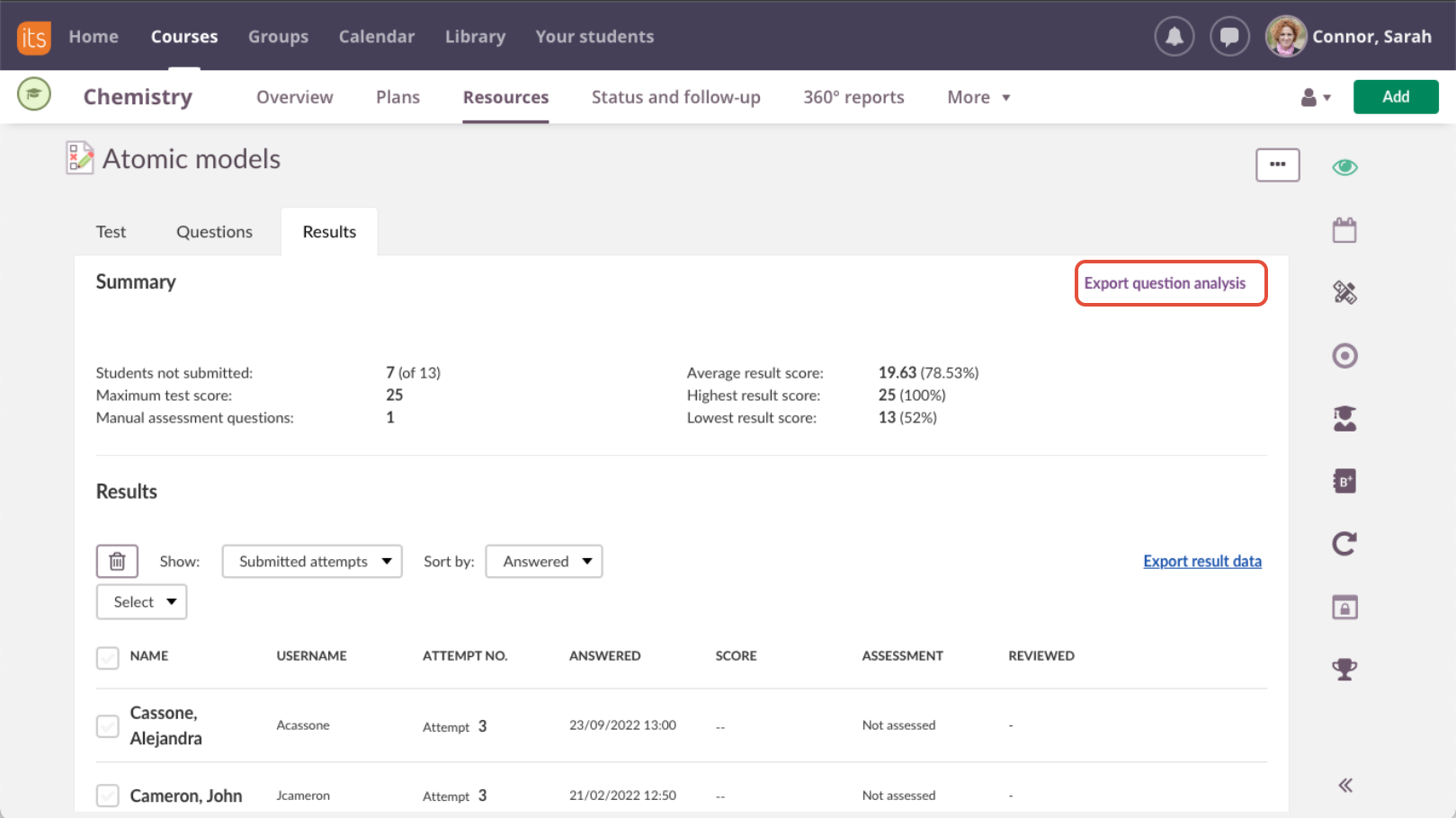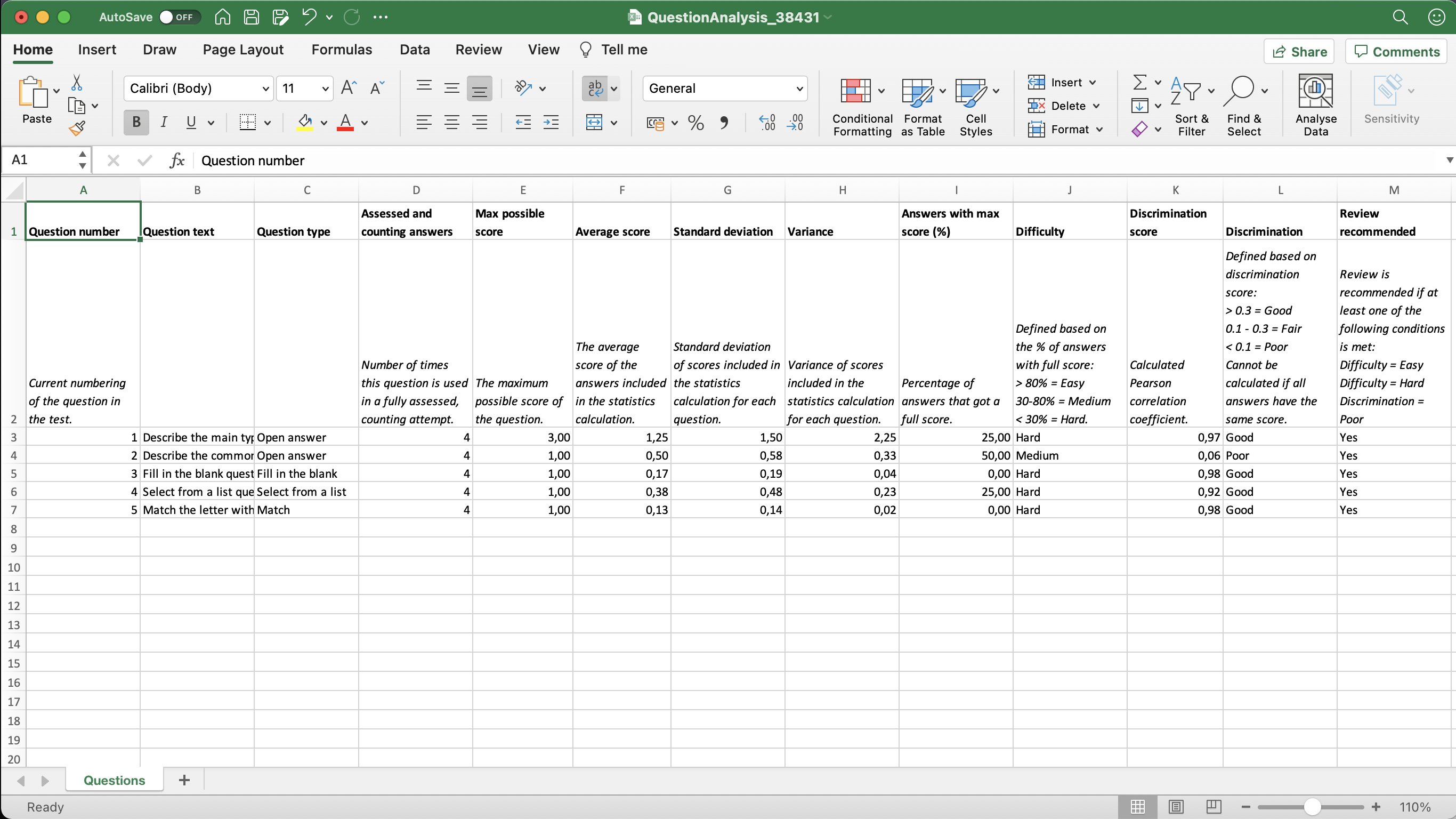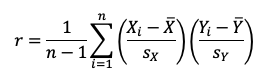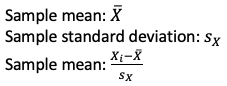# Introduction

For high stakes tests or exams, in particular in higher education, teachers want to analyse the quality of their questions. There are established methods for this, looking at the difficulty and discrimination of each question based on the student answers. This is not something that we have had in itslearning before, and we are happy to announce that such an analysis is now available. The option is found in the Results tab, close to the summary information for the test. Simply press "Export question analasys". This triggers the calculation and subsequent download of an excel (.xsls) file. The analysis is performed on all counting attempts that are fully assessed (max one per student). If a student later submits a new attempt that becomes the counting attempt, simply click the button again to trigger a new analysis.The excel spreadsheet will have a header row, followed by a row with a short descriptions of what each column contains. There is one row for each question in the test.Further column classification:

• ### Explanations for each column

Column
Possible values
Explanation
Column
Possible values
Explanation
Question numberIntegersQuestion number as seen in the question list in the test by the teacher.
Question textUp to 100 characters of text

Question text, cut at 100 characters if long. HTML is stripped.

For Fill in the blank or Select from a list, this is the introduction text, not the question.

Question typeTextName of the question type (currently 7 available)
Assessed and counting answersIntegersNumber of times the questions has been used in a fully assessed, counting attempt.
Max possible scorePositive number, 2 decimalsQuestion score as set by the teacher when creating the question. Usually an integer, but decimal values are allowed.
Average scorePositive number, 2 decimalsAverage score achieved in the attempts included in the analysis.
Standard deviationPositive number, 2 decimalsStandard deviation of the scores achieved in the attempts included in the analysis. Calculated as standard deviation for a sample population.
VariancePositive number, 2 decimalsVariance of the scores achieved in the attempts included in the analysis. Calculated as variance for a sample population.
Answers with max score (%)Positive number, 2 decimalsPercentage of attempts with score = max score, based on the number of attempts included in the analysis.
DifficultyHard, medium or easy

The difficulty is based on the percentage of attempts which achieved the max score, and categorised as follows:

> 80% = Easy (meaning more than 80% of students got this correct)
30-80% = Medium
< 30% = Hard (meaning less than 30% of students got this correct)

Discrimination scorePositive number between 0 and 1, 2 decimals, or empty.

The discrimination score is calculated as Pearson's correlation coefficiant for a sample population. The coefficient cannot be calculated in cases where all attempts have the same score.

The formula for this isWhereGood, fair, poor or cannot calculate

The discrimination is based on the discrimination score, and categorised as follows:

> 0.3 = Good
0.1 - 0.3 = Fair
< 0.1 = Poor

Review recommendedYes or no

Based on the difficulty and discrimination values, we will recommend review of the question if at least one of the following conditions is met:

Difficulty = Easy
Difficulty = Hard
Discrimination = Poor

Did you find it helpful? Yes No

Send feedback